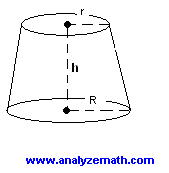# Find The Volume of a Frustum Using Calculus

Find the volume of a frustum using integrals and calculus.

 Problem : Find the volume of a frustum with height h and radii r and R as shown below.Solution to the problem: A frustum may be obtained by revolving y = x between x = a and x = b around the x axis as shown below. The height h = b - a. The volume of the frustum obtained is given byVolume =x1x2p [ f(x) ] 2 dx =abp [ x 2] dy = p [ x 3 / 3] ab = p [ b 3 / 3 - a 3 / 3] We now factor the term b 3 - a 3 and rewrite the expression for the volume as follows Volume = p / 3 [ (b - a)(b 2 + a b + a 2 ] We now substitute the following: h = b - a and y = x gives r = a and R = b into the expression of the volume to obtain a formula for the volume of the frustum Volume = p / 3 [ h (R 2 + r R + r 2 ] More references on integrals and their applications in calculus.